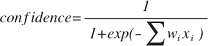# 16.20 - SVMSparsePredict Output - Teradata Database - Teradata Vantage NewSQL Engine

## Teradata Vantage™ - NewSQL Engine Analytic Functions

Product
Release Number
16.20
Release Date
July 2019
Content Type
Programming Reference
Publication ID
B035-1206-162K
Language
English (United States)

## Output Table Schema

The table has the predicted class of each test sample.

Column Data Type Description
id_column INTEGER, SMALLINT, BIGINT, NUMERIC, NUMERIC(p), NUMERIC(p,a), VARCHAR, or VARCHAR(n) Test sample identifier.
predict_value VARCHAR Predicted class of test sample.
predict_confidence DOUBLE PRECISION Prediction confidence, a value between 0 and 1, computed by formula that follows this table. The higher the value, the more dependable the prediction.
accumulate_column Any [Column appears once for each specified accumulate_column.] Column copied from InputTable.

## Formula for predict_confidencewhere i is the attribute id, x i is the value of attributes in the sample, and w i is the weight of attribute i.